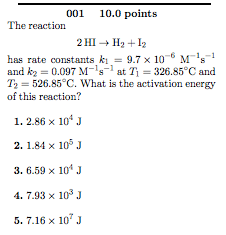# The reaction 2 HI → H2 + I2 has the rate constant k1 = 9.7x10^-6 M^-1 s^-1 and k2 = 0.097 M^-1 s^-1 at T1 = 326.85 degrees C and T2 = 526.85 degrees C. What is the activation energy of this reaction? 1. 2.86x10^4 J 2. 1.84x10^5 J 3. 6.59x10^4 J 4. 7.93x10^3 J 5. 7.16x10^7 J